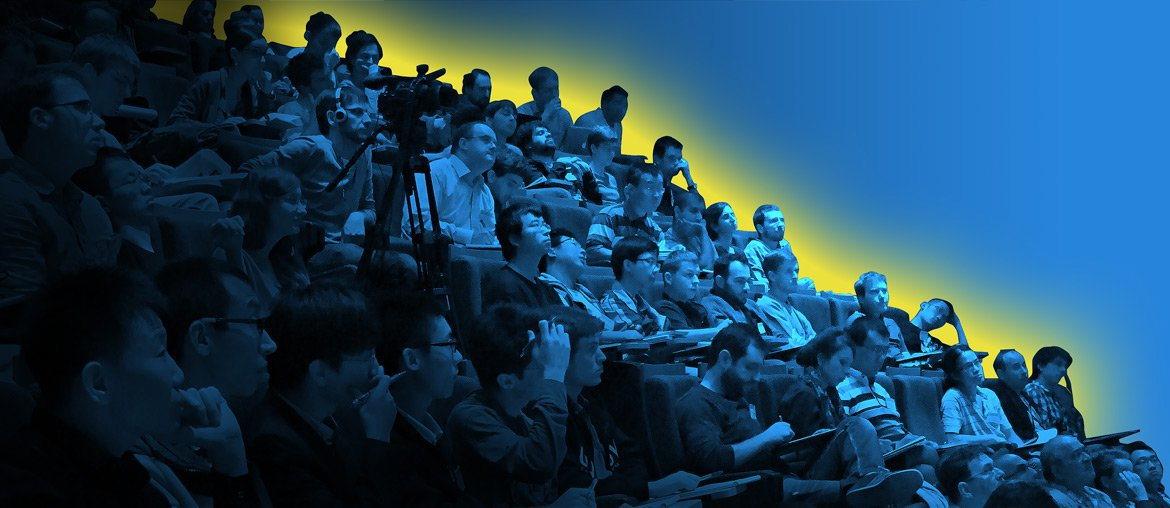• Accueil

• La FMJH Organise

• Evénements

# 2019-2022

## 2022 Pr Corinna Ulcigrai

### "Parabolic dynamics and renormalization: an introduction"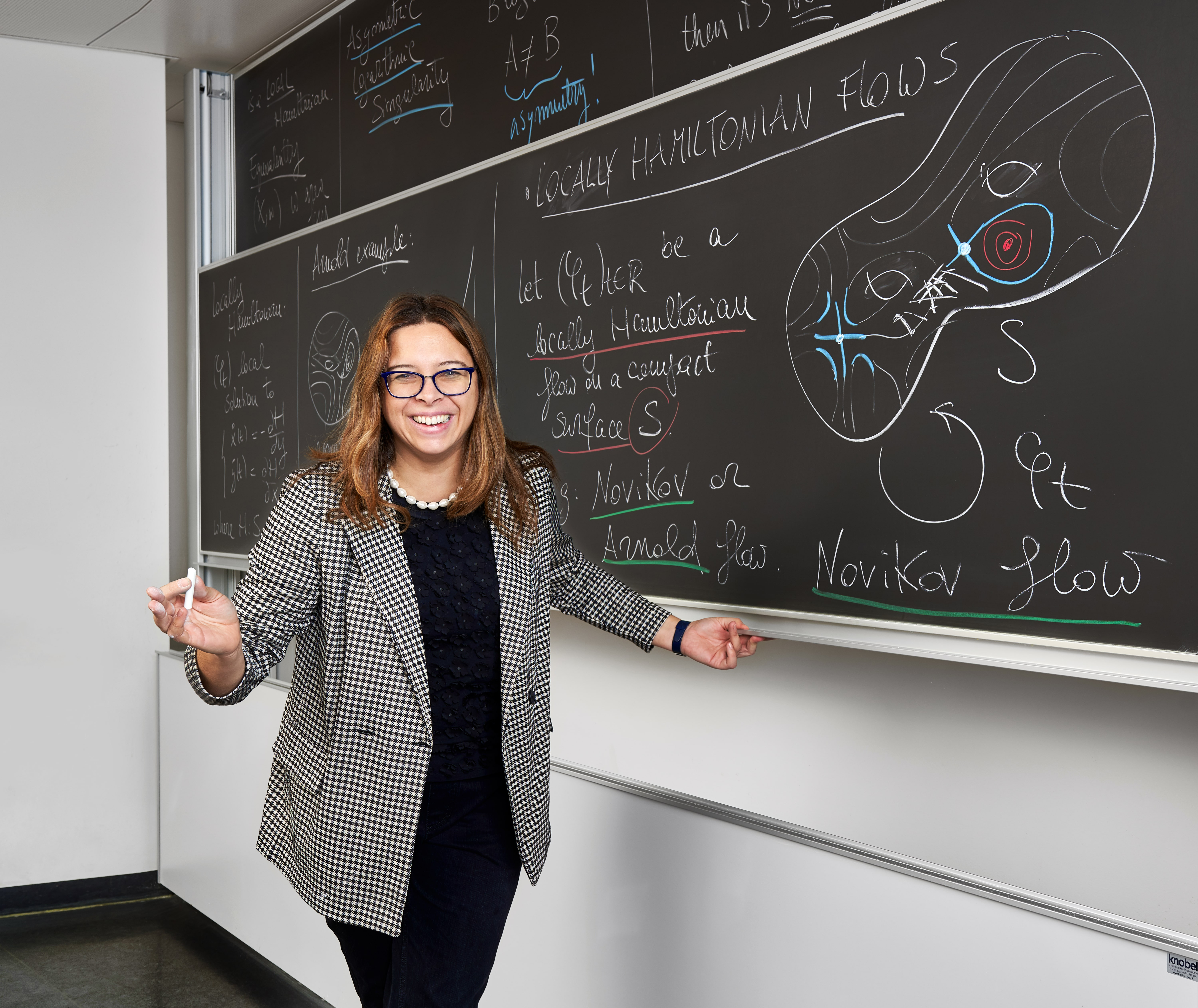Parabolic dynamical systems are mathematical models of the many phenomena which display a "slow" form of chaotic evolution, in the sense that nearby trajectories diverge polynomially in time.
In contrast with hyperbolic and elliptic dynamical systems, there is no general theory which describes parabolic dynamics. In recent years, a lot of progress has been done in understanding the chaotic features of several classes of such systems, as well as in identifying key mechanisms and techniques which play a central role in their study.
In these lectures we will give a self-contained introduction to some results on chaotic features of parabolic flows, some classical as well as many very recent. We will in particular discuss:
-renormalizable linear flows;
-horocycle flows on compact hyperbolic surfaces and their time-changes;
-the Heisenberg nilflow, nilflows on nilmanifolds and their time-changes;
-smooth area preserving (also known as 'locally Hamiltonian') flows on surfaces.
We will define the mathematical objects as well as the dynamical properties we will discuss to keep the lectures accessible to a wide audience and try to highlight throughout the importance of phenomena such as shearing and techniques based on renormalization. Connections between parabolic flows and mathematical physics, spectral theory and Teichmueller dynamics will also be mentioned.

## 2021 Pr Karim Alexander Adiprasito

### "Lefschetz, Hodge and combinatorics: an account of a fruitful cross-pollination"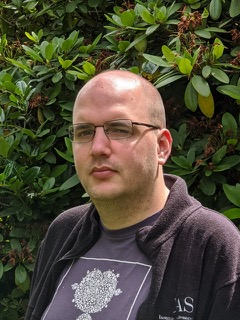Almost 40 years ago, Stanley noticed that some of the deep theorems of algebraic geometry have powerful combinatorial applications. Among other things, he used the hard Lefschetz theorem to rederive Dynkin's theorem, and to characterize face numbers of simplicial polytopes.

Since then, several more deep combinatorial and geometric problems were discovered to be related to theorems surrounding the Lefschetz theorem. One first constructs a ring metaphorically modelling the combinatorial problem at hand, often modelled on constructions for toric varieties, and then tries to derive the combinatorial result using deep results in algebraic geometry. For instance

- a Lefschetz property for implies that a simplicial complex PL-embedded in R^{4} cannot have more triangles than four times the number of it's edges (Kalai/A.),

- a Hodge-Riemann type property implies the log-concavity of the coefficients of the chromatic polynomial. (Huh),

- a decomposition type property implies the positivity of the Kazhdan-Lusztig polynomial (Elias-Williamson),

At this point one can then hope that indeed, algebraic geometry provides the answer, which is often only the case in very special cases, when there is a sufficiently nice variety behind the metaphor.

It is at this point that purely combinatorial techniques can be attempted to prove the desired. This is the modern approach to the problem, and I will discuss the two main approaches used in this area: Firstly, an idea of Peter McMullen, based on local modifications of the ring and control of the signature of the intersection form. Second, an approach based on a theorem of Hall, using the observation that spaces of low-rank linear maps are of special form.

Attention : En raison des nouvelles restrictions liées à la COVID-19, nous sommes malheureusement dans l’obligation d’organiser les leçons Hadamard 2021 en ligne.

## 2020 Pr Vlad Vicol and Pr Tristan Buckmaster

### « Wild weak solutions to equations arising in hydrodynamics »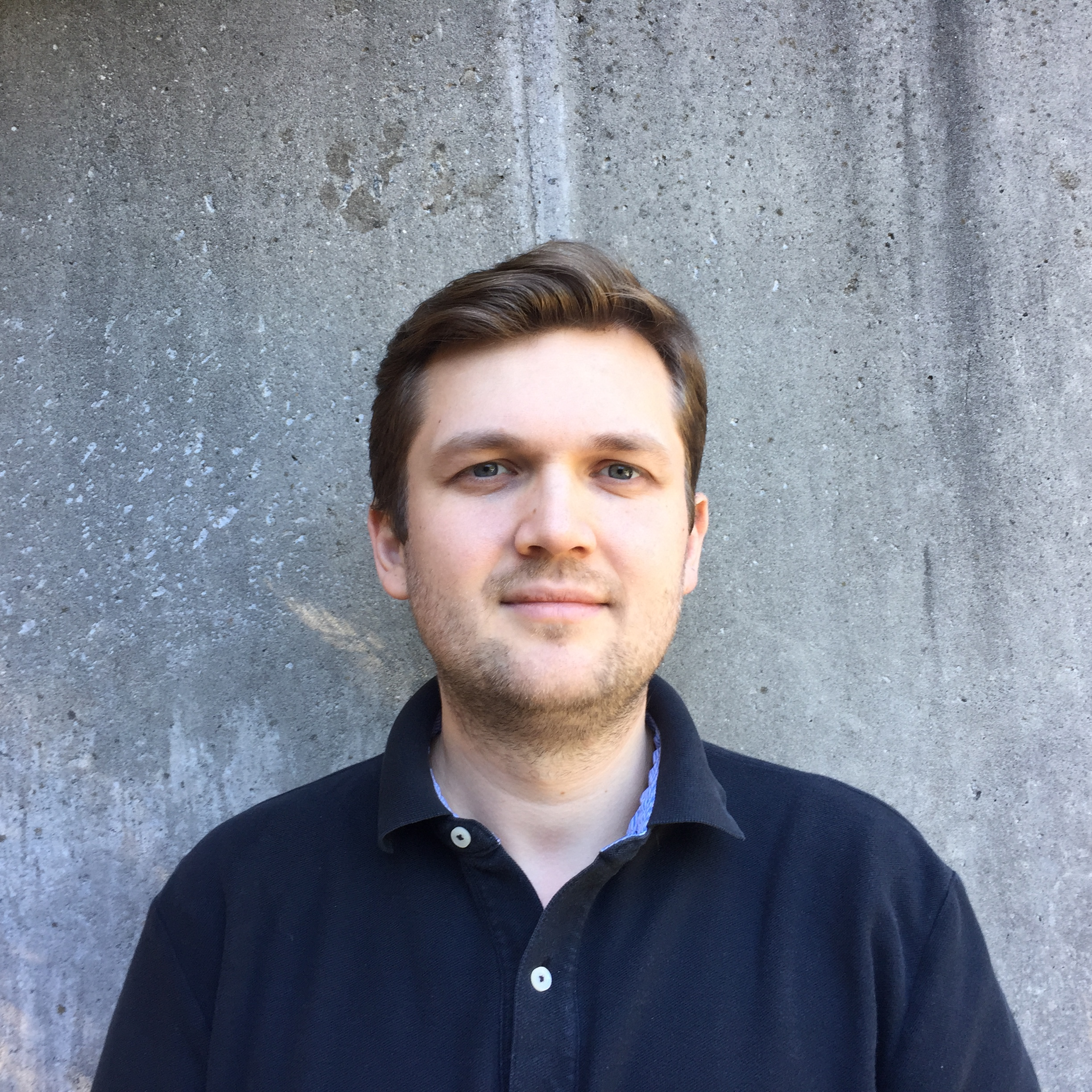In this course, we will discuss the use of convex integration to construct wild weak solutions in the context of the Euler and Navier-Stokes equations. In particular, we will outline the resolution of Onsager’s conjecture as well as the recent proof of non-uniqueness of weak solutions to the Navier-Stokes equations. Onsager’s conjecture states that weak solutions to the Euler equation belonging to Hölder spaces with Hölder exponent greater than 1/3 conserve energy, and conversely, there exist weak solutions lying in any Hölder space with exponent less than 1/3 which dissipate energy. The conjecture itself is linked to the anomalous dissipation of energy in turbulent flows, which has been called the zeroth law of turbulence. For initial datum of finite kinetic energy, Leray has proven that there exists at least one global in time finite energy weak solution of the 3D Navier-Stokes equations. We prove that weak solutions of the 3D Navier-Stokes equations are not unique, within a class of weak solutions with finite kinetic energy. The non-uniqueness of Leray-Hopf solutions is the subject of a famous conjecture of Ladyženskaja in ‘69, and to date, this conjecture remains open.

En raison de la crise sanitaire, les leçons du Pr Tristan Buckmaster ont été annulées.

## 2019 Maryna Viazovska

### «Automorphic forms and optimization in Euclidean space»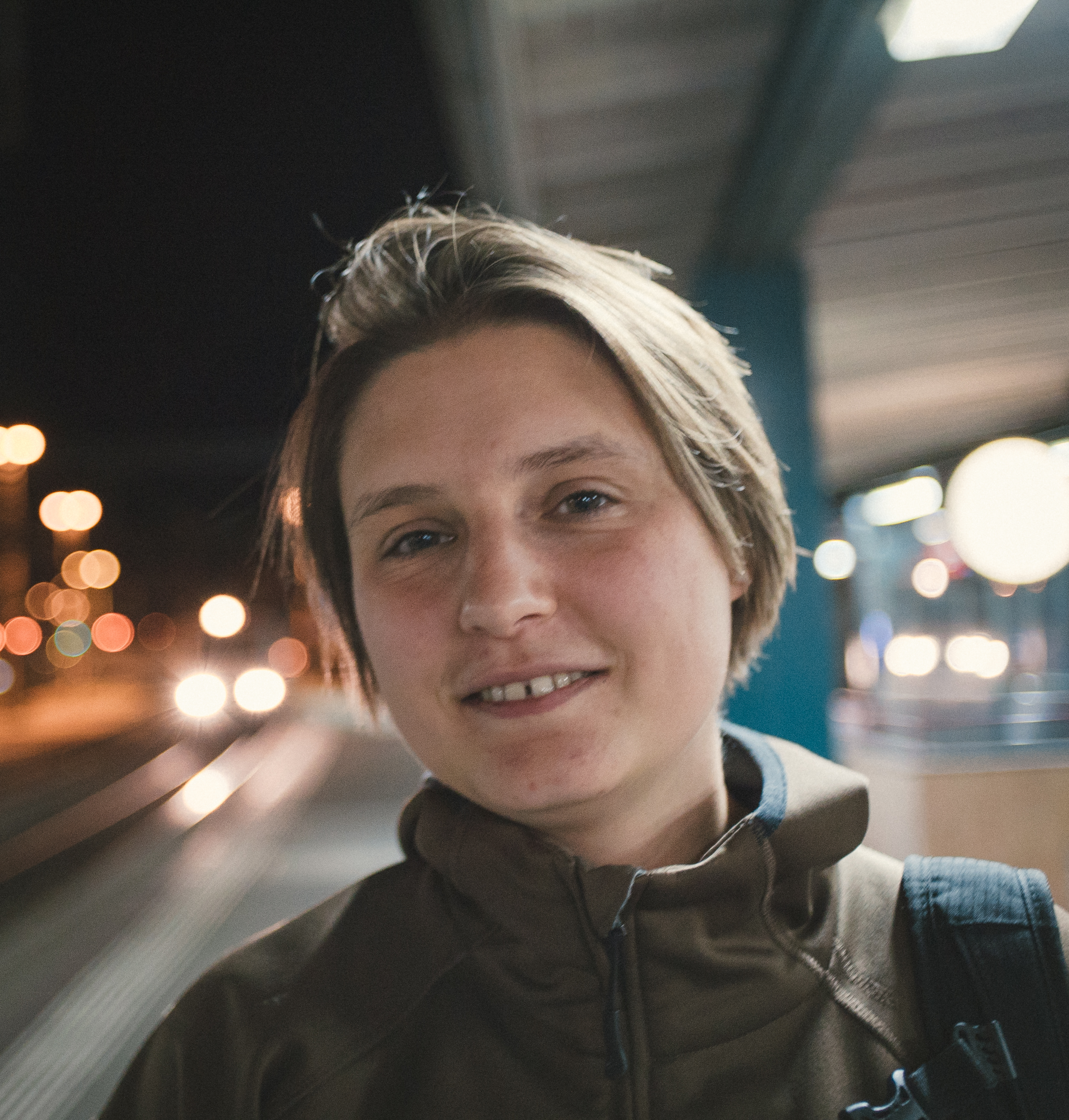The goal of this lecture course is to prove the universaloptimality of the E8 and Leech lattices.

This theorem is the main result of a recent preprint «Universal optimality of the E8 and Leech lattices and interpolation formulas» written in collaboration with Henry Cohn, Abhinav Kumar, Stephen D. Miller and Danylo Radchenko. We prove that the E8 and Leech lattices minimize energy of every potential function that is a completely monotonic function of squared distance (for example, inverse power laws or Gaussians).

This theorem implies recently proven optimality of E8 and Leech lattices as sphere packings and broadly generalizes it to long-range interactions. The key ingredient of the proof is sharp linear programming bounds. To construct the optimal auxiliary functions attaining these bounds, we prove a new interpolation theorem.

At the last lecture, we will discuss open questions and conjectures which arose from our work.